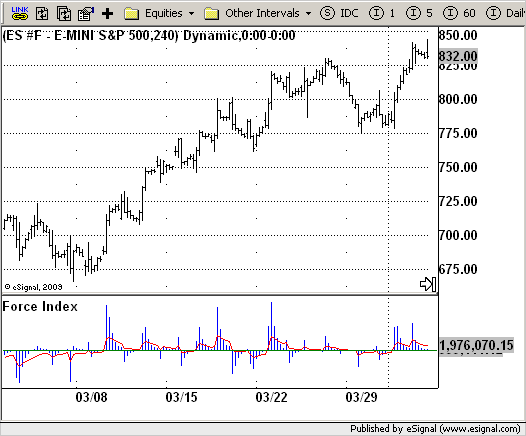# Force Index

ICE Data Services -

ForceIndex.efs

File Name: ForceIndex.efs

Description:
Force Index

Formula Parameters:

• XLen1 : 3
• XLen3 : 13

Notes:
This Indicator plots the Force Index as described by Dr. Alexander Elder in "Trading For a Living." The ForceIndex indicator relates price to volume by multiplying net change and volume. ForceIndex is calculated using the following equation:

• ForceIndex = Volume(today) * (Close(this period) - Close(last period))
• ForceIndex is typically presented as two smoothed averages (slow and fast) to avoid false signals.

ForceIndex.efsEFS Code:

```/*********************************
Provided By:
eSignal (Copyright c eSignal), a division of Interactive Data
Formula Script (EFS) is for educational purposes only and may be
modified and saved under a new file name.  eSignal is not responsible
for the functionality once modified.  eSignal reserves the right
to modify and overwrite this EFS file with each new release.

Description:
Force Index

Version:            1.0  04/15/2009

Formula Parameters:                     Default:
XLen1                               3
XLen3                               13
Notes:
This Indicator plots the Force Index as described by Dr. Alexander
Elder in "Trading For a Living." The ForceIndex indicator relates
price to volume by multiplying net change and volume. ForceIndex is
calculated using the following equation:
ForceIndex = Volume(today) * (Close(this period) - Close(last period))
ForceIndex is typically presented as two smoothed averages (slow and fast)
to avoid false signals.
**********************************/
var fpArray = new Array();
var bInit = false;

function preMain() {
setStudyTitle("Force Index");
setCursorLabelName("FastAvg", 0);
setCursorLabelName("SlowAvg", 1);
setDefaultBarFgColor(Color.blue, 0);
setDefaultBarFgColor(Color.red, 1);
setPlotType(PLOTTYPE_HISTOGRAM, 0);
var x = 0;
fpArray[x] = new FunctionParameter("XLen1", FunctionParameter.NUMBER);
with(fpArray[x++]) {
setLowerLimit(1);
setDefault(3);
}
fpArray[x] = new FunctionParameter("XLen2", FunctionParameter.NUMBER);
with(fpArray[x++]) {
setLowerLimit(1);
setDefault(13);
}
}

var xForce1 = null;
var xForce2 = null;

function main(XLen1, XLen2) {
var nBarState = getBarState();
var nForce1 = 0;
var nForce2 = 0;
if (nBarState == BARSTATE_ALLBARS) {
if (XLen1 == null) XLen1 = 3;
if (XLen2 == null) XLen2 = 13;
}

if (bInit == false) {
xForce1 = efsInternal("Calc_Force_Index", XLen1, XLen2);
xForce2 = getSeries(xForce1, 1);
bInit = true;
}
nForce1 = xForce1.getValue(0);
nForce2 = xForce2.getValue(0);

return new Array(nForce1, nForce2);
}

var xValue = null;
var xSMA1 = null;
var xSMA2 = null;
var bSecondInit = false;

function Calc_Force_Index(nLen1, nLen2) {
var nRes = 0;
var nSMA1 = 0;
var nSMA2 = 0;
if (bSecondInit == false) {
xValue = efsInternal("Calc_Value");
xSMA1 = ema(nLen1, xValue);
xSMA2 = ema(nLen2, xValue);
bSecondInit = true;
}
nSMA1 = xSMA1.getValue(0);
nSMA2 = xSMA2.getValue(0);
if (nSMA1 == null || nSMA2 == null) return;
return new Array(nSMA1, nSMA2);
}

function Calc_Value() {
var nRes = 0;
nRes = volume(0) * (close(0) - close(-1));
if (nRes == null) return;
return nRes;
}```